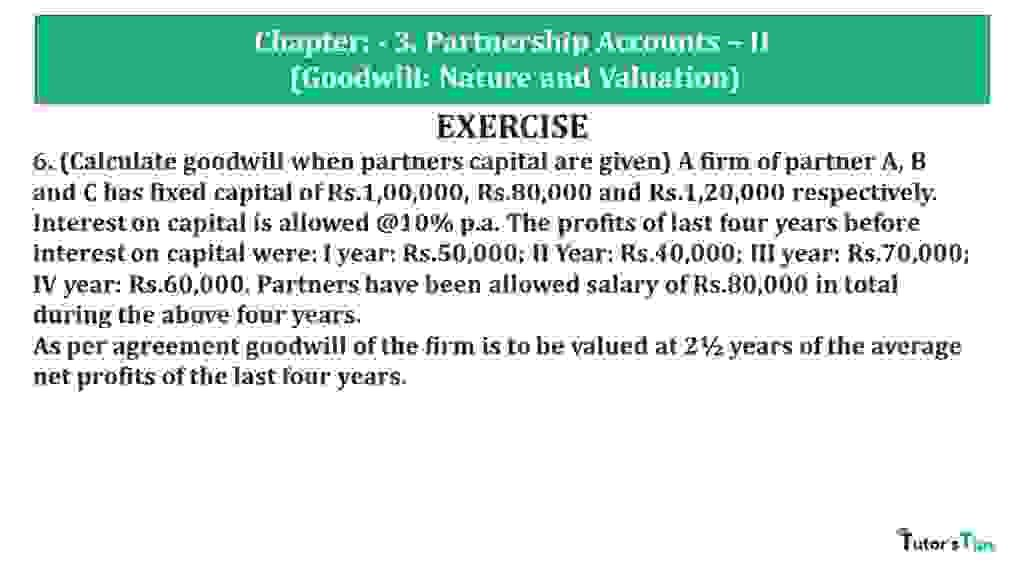# Question 06 Chapter 3 of +2 Part-1 – USHA Publication 12 Class Part – 1Question 06 Chapter 3 of +2-Part-1

6. (Calculate goodwill when partners capital are given) A firm of partner A, B and C has fixed capital of Rs.1,00,000, Rs.80,000 and Rs.1,20,000 respectively. Interest on capital is allowed @10% p.a. The profits of last four years before interest on capital were: I year: Rs.50,000; II Year: Rs.40,000; III year: Rs.70,000; IV year: Rs.60,000. Partners have been allowed salary of Rs.80,000 in total during the above four years.
As per agreement goodwill of the firm is to be valued at 2½ years of the average net profits of the last four years.

### The solution of Question 06 Chapter 3 of +2 Part-1: –

 Average Profit = Total Profit for past given years Number of years = 20,000 + 10,000 + 40,000 + 30,000 4 = 1,00,000 3 = 25,000

 Number of years’ purchase = 2 Goodwill = Average Profit X Number of years’ purchase Goodwill = 25,000 X 2.5 Goodwill = 62,500

Working Note : –

*1 Calculation of Profits of last three years

 Adjusted Profit for 1st year = Total Profit −Interest on capital = 50,000 –(10,000+8,000+12,000) = 20,000

 Adjusted Profit for 2nd year = Total Profit – Interest on capital = 40,000 –(10,000+8,000+12,000) = 10,000

 Adjusted Profit for 3rd year = Total Profit − Interest on capital = 70,000 –(10,000+8,000+12,000) = 40,000

 Adjusted Profit for 3rd year = Total Profit − Interest on capital = 60,000 –(10,000+8,000+12,000) = 30,000

Every year, interest on capital will be deducted amounting to Rs.3,000 for four years.